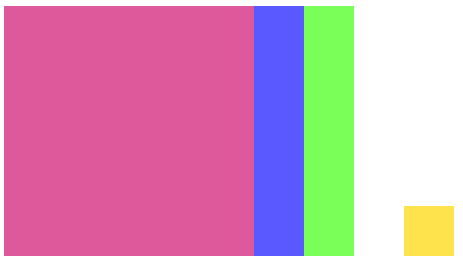You may also likeSumming Consecutive Numbers

15 = 7 + 8 and 10 = 1 + 2 + 3 + 4. Can you say which numbers can be expressed as the sum of two or more consecutive integers?Always the Same

Arrange the numbers 1 to 16 into a 4 by 4 array. Choose a number. Cross out the numbers on the same row and column. Repeat this process. Add up you four numbers. Why do they always add up to 34?Fibs

The well known Fibonacci sequence is 1 ,1, 2, 3, 5, 8, 13, 21.... How many Fibonacci sequences can you find containing the number 196 as one of the terms?

Age 11 to 14Challenge Level

Charlie has been playing with calculations again...

$2 \times 4 + 1 = 9$
$4 \times 6 + 1 = 25$
$5 \times 7 + 1 = 36$
$9 \times 11 + 1 = 100$

What do you notice?

Click below to see what Charlie said:

"If you multiply two numbers that differ by 2, and then add one, the answer is always the square of the number between them!"

Can you explain what's happening?

Click below to see Charlie's explanation:

$n(n+2) +1 = n^2 +2n+1 = (n+1)^2$
or alternatively,
$(n-1)(n+1)+1 = n^2-n+n-1+1=n^2$

Alison drew a diagram to explain the results. Click below to see:Can you make sense of Charlie's method and Alison's diagrams?

Here are some more number patterns to explore. Some have been expressed numerically, some in words, and some algebraically.

Can you represent each pattern in all four ways,

numerically (so you can spot the pattern),
in words (so you can describe the pattern),
algebraically (so you can prove the pattern continues),
and using a diagram (to explain the pattern)?

1. $2 \times 3 + 3 = \, ?$
$5 \times 6 + 6 = \, ?$
$4 \times 5 + 5 = \, ?$
$9 \times 10 + 10 = \, ?$
What do you notice?

2. Choose three consecutive numbers, square the middle one, and subtract the product of the other two.
Repeat with some other sets of numbers.
What do you notice?

3. $3 \times 3 - 1 \times 1 = \, ?$
$8 \times 8 - 6 \times 6 = \, ?$
$7 \times 7 - 5 \times 5 = \, ?$
$10 \times 10 - 8 \times 8 = \, ?$
What do you notice?

4. $n(n+1) - (n-1)(n+2) = \, ?$
$(n+1)(n+2) - n(n+3) = \, ?$
$(n-3)(n-2) - (n-4)(n-1) = \, ?$
What do you notice?

5. $3\times 5 + 1= \, ?$
$5\times 7 + 1= \, ?$
$7\times 9 + 1= \, ?$
$9\times 11 + 1= \, ?$
What do you notice?

6. Choose three consecutive numbers and add the product of the smallest two to the product of the greatest two.
Repeat with some other sets of numbers.
What do you notice?

With thanks to Don Steward, whose ideas formed the basis of this problem.

You may be interested in the other problems in our Factorise This! Feature.